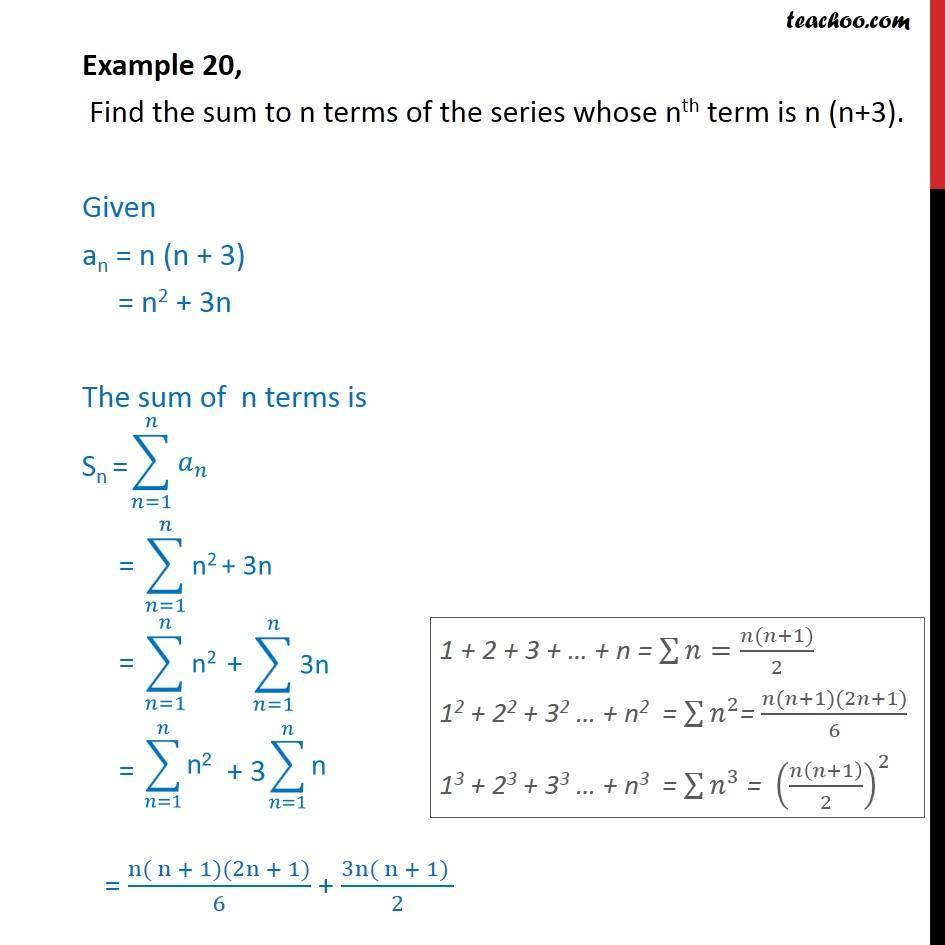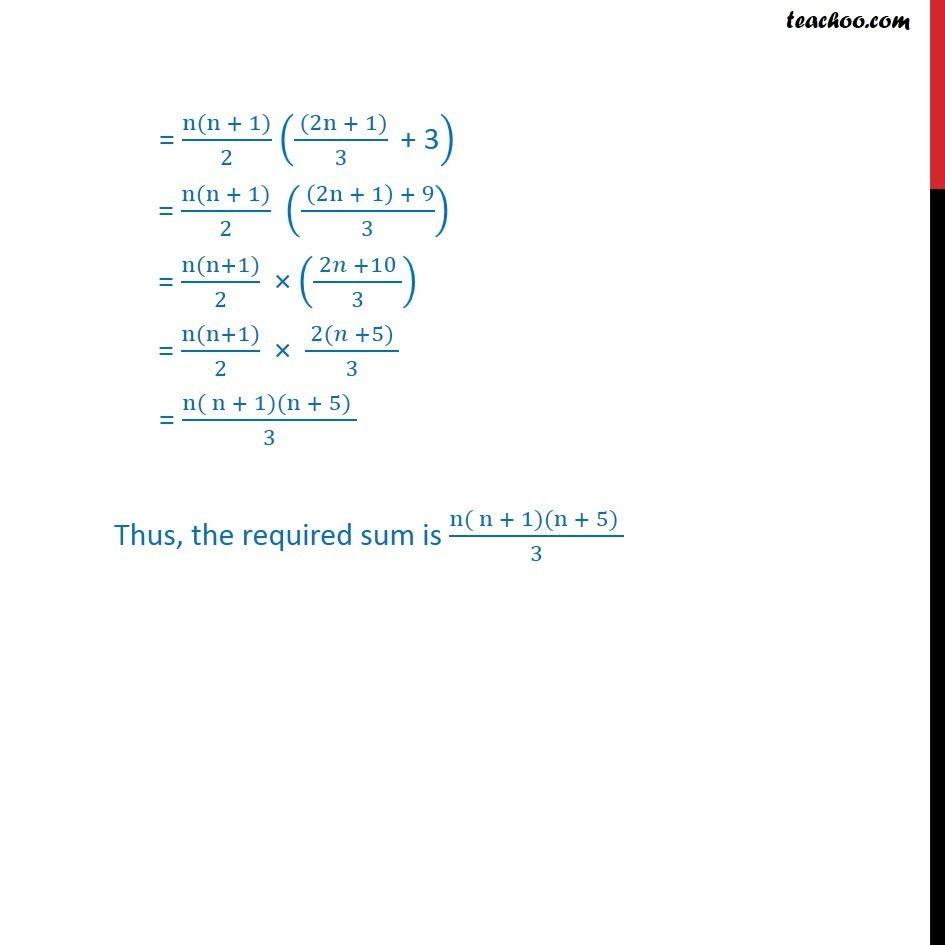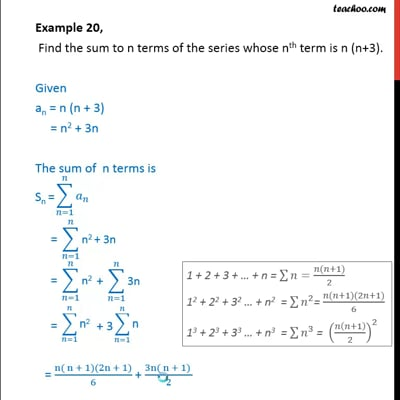Examples

Chapter 9 Class 11 Sequences and Series (Term 1)
Serial order wiseThis video is only available for Teachoo black users

### Transcript

Example 20, Find the sum to n terms of the series whose nth term is n (n+3). Given an = n (n + 3) = n2 + 3n The sum of n terms is = (n( n + 1)(2n + 1))/6 + (3n( n + 1) )/2 = (n(n + 1))/2 (( (2n + 1))/3 " + 3" ) = (n(n + 1))/2 (( (2n + 1) + 9)/3) = (n(n+1))/2 × (( 2𝑛 +10 )/3) = (n(n+1))/2 × ( 2(𝑛 +5) )/3 = (n( n + 1)(n + 5) )/3 Thus, the required sum is (n( n + 1)(n + 5) )/3